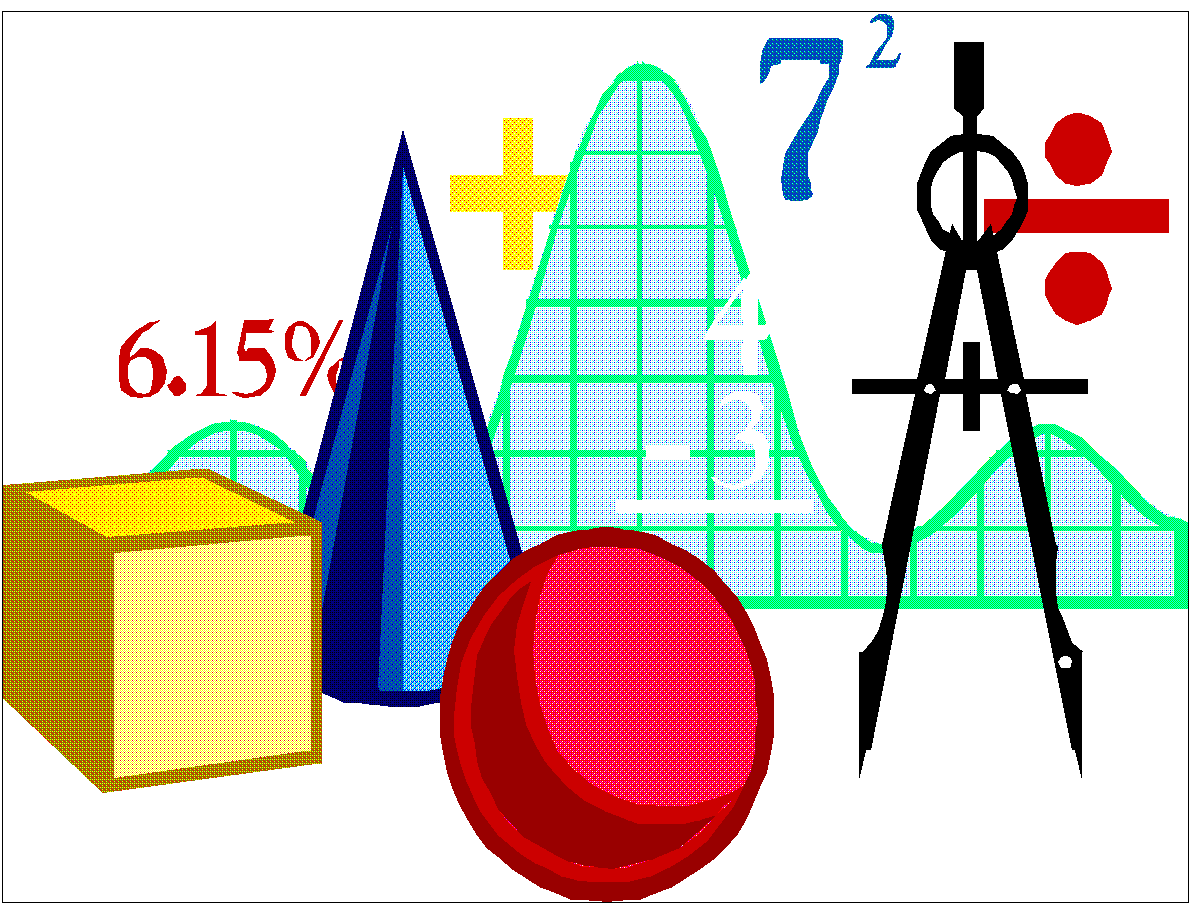# Calculus with Applications A/B

`Prerequisite: PrecalculusCalculus with Applications topics include limits, continuity, and derivatives of functions, the definite integral, and their real-world applications. Students find and apply derivatives numerically, graphically, and symbolically. Students will analyze previously studied functions using calculus concepts. The relationship between the derivative and the definite integral is developed. Students will model real-world situations involving rates of change using difference or differential equations.`

Precalculus